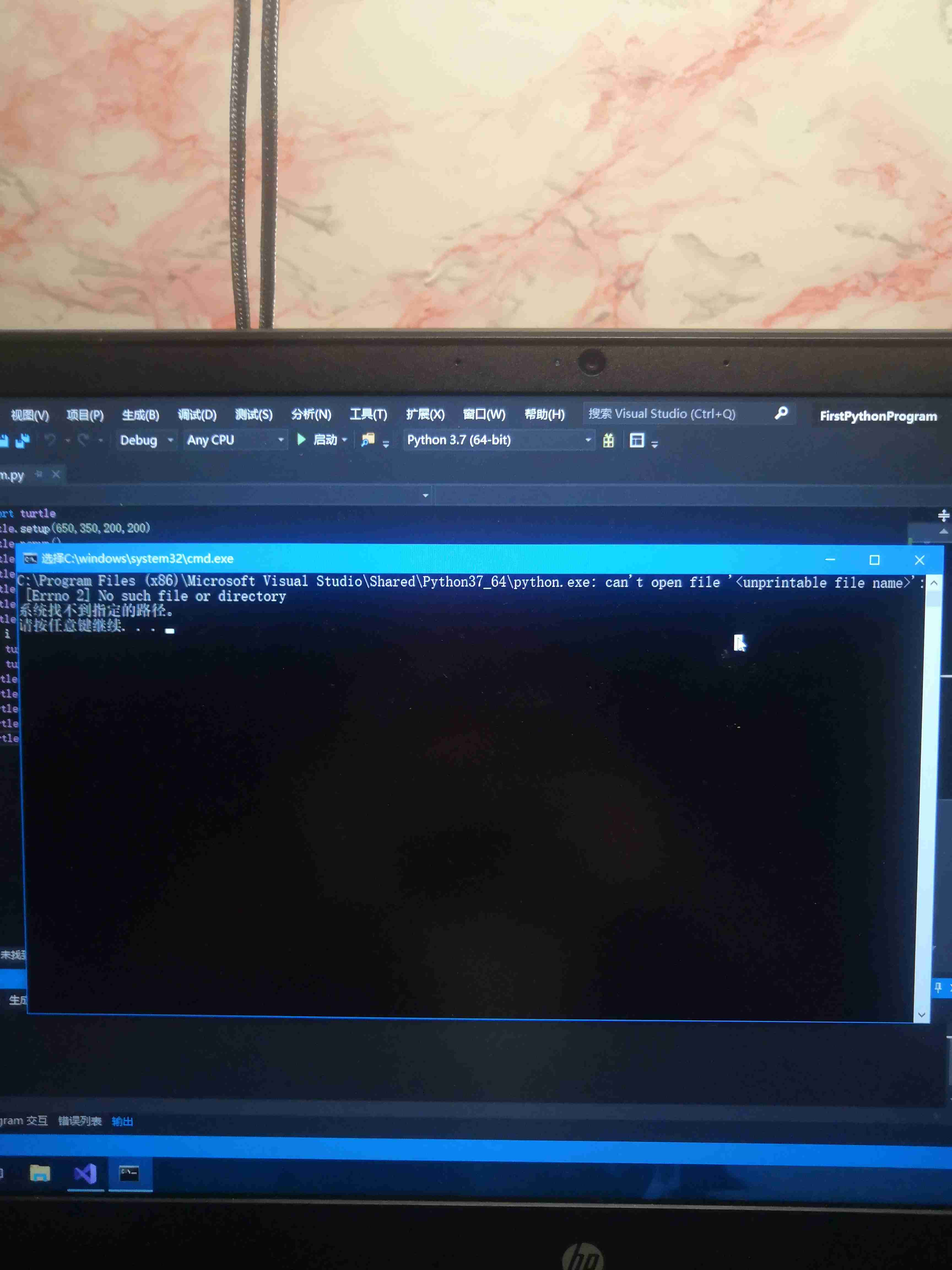vs 运行python代码，控制台说找不到路径 5C1个回答

python 的环境变量,或者python安装位置错误python 控制台输出的异常捕获不到

Visual Code 运行python代码报错

python中如何将控制台数据保存到mysql中

vs 运行python 环境变量怎样配置

python爬虫运行后找不到爬取的图片

#!/usr/bin/python # -*- coding: UTF-8 -*- import re import urllib.request def getHtml(url): page=urllib.request.urlopen(url) html=page.read() return html html=getHtml("http://tieba.baidu.com/p/3205263090") html=html.decode('UTF-8') def getImg(html): reg=r'src="(.+?\.jpg)"pic_ext' imgre=re.compile(reg) imgList=re.findall(imgre,html) return imgList imgList=getImg(html) imgName=0 for imgPath in imgList: f=open("F:/pic/"+str(imgName)+".jpg",'wb') f.write((urllib.request.urlopen(imgPath)).read()) f.close() imgName+=1 print("all done") 代码如上，在f盘的pic文件夹下找不到图片。

vscode运行python代码出现运行不了货者“调试适配器进程已意外终止”怎么办？

Python绘制词频统计图，怎么输出到GUI，而不是输出到控制台？

# -*- coding: utf-8 -*- from tkinter import * import tkinter import hashlib import time import codecs import codecs import matplotlib.pyplot as plt from pylab import mpl mpl.rcParams['font.sans-serif'] = ['FangSong'] # 指定默认字体 mpl.rcParams['axes.unicode_minus'] = False # 解决保存图像是负号'-'显示为方块的问题 LOG_LINE_NUM = 0 class MY_GUI(): def __init__(self,init_window_name): self.init_window_name = init_window_name #设置窗口 def set_init_window(self): self.init_window_name.title("维吉尼亚密码工具") #窗口名 self.init_window_name.geometry('320x160+10+10') #290 160为窗口大小，+10 +10 定义窗口弹出时的默认展示位置 self.init_window_name.geometry('1000x680+10+10') self.init_window_name["bg"] = "white" #窗口背景色，其他背景色见：blog.csdn.net/chl0000/article/details/7657887 self.init_window_name.attributes("-alpha",1.0) #虚化，值越小虚化程度越高 self.init_data_label = Label(self.init_window_name, text="待处理数据") self.init_data_label.grid(row=0, column=0) self.result_data_label = Label(self.init_window_name, text="输出结果") self.result_data_label.grid(row=0, column=15) self.init_key_label = Label(self.init_window_name, text="密钥") self.init_key_label.grid(row=0, column=10) self.log_label = Label(self.init_window_name, text="日志") self.log_label.grid(row=12, column=0) self.init_data_Text = Text(self.init_window_name, width=50, height=35) # 原始数据录入框 self.init_data_Text.grid(row=1, column=0, rowspan=10, columnspan=10) self.result_data_Text = Text(self.init_window_name, width=35, height=49) # 处理结果展示 self.result_data_Text.grid(row=1, column=12, rowspan=15, columnspan=10) self.key_Text = Text(self.init_window_name, width=5, height=1) # 密钥框 self.key_Text.grid(row=1, column=6, rowspan=1,columnspan=10) self.log_data_Text = Text(self.init_window_name, width=66, height=9) # 日志框 self.log_data_Text.grid(row=13, column=0, columnspan=10) #按钮 self.str_transtoencrypt_button = Button(self.init_window_name, text="加密", bg="lightblue", width=10,command=self.str_transtoencrypt) # 调用内部方法 加()为直接调用 self.str_transtoencrypt_button.grid(row=3, column=11) self.str_transtodecrypt_button = Button(self.init_window_name, text="解密", bg="lightblue", width=10,command=self.str_transtodecrypt) # 调用内部方法 加()为直接调用 self.str_transtodecrypt_button.grid(row=5, column=11) self.str_count_button = Button(self.init_window_name, text="词频统计", bg="lightblue", width=10,command=self.str_count) # 调用内部方法 加()为直接调用 self.str_count_button.grid(row=7, column=11) #功能算法的实现 #加密算法 def str_transtoencrypt(self): letter_list = 'ABCDEFGHIJKLMNOPQRSTUVWXYZ'; src = self.init_data_Text.get(1.0,END).strip().replace("\n","") #获得输入的字符 key = self.key_Text.get(1.0, END).strip().replace("\n", "") #获得输入的密钥 a=[] for ch in key: a.append(ord(ch.upper())-65) #ord()函数返回ASCii值 upper()将小写转大写Python 列表 append() 方法用于在列表末尾追加新的对象。 get_list = a ciphertext = ''; i = 0 for ch in src: # 遍历明文 if i % len(get_list) == 0: i = 0 if ch.isupper(): # isupper函数检测字符串中是否都是大写字母 ciphertext += letter_list[(ord(ch) - 65 + get_list[i]) % 26] i += 1 else: ciphertext += letter_list[(ord(ch) - 97 + get_list[i]) % 26].lower() i += 1 self.result_data_Text.delete(1.0, END) self.result_data_Text.insert(1.0, ciphertext) #解密算法 def str_transtodecrypt(self): letter_list = 'ABCDEFGHIJKLMNOPQRSTUVWXYZ'; src = self.init_data_Text.get(1.0, END).strip().replace("\n", "") # 获得输入的字符 key = self.key_Text.get(1.0, END).strip().replace("\n", "") # 获得输入的密钥 a=[] for ch in key: a.append(ord(ch.upper())-65) #ord()函数返回ASCii值 upper()将小写转大写Python 列表 append() 方法用于在列表末尾追加新的对象。 get_list = a ciphertext = ''; i = 0 plaintext=''; i = 0 for ch in src: #遍历密文 if i%len(get_list)==0: i=0 #判断是否是大写 if ch.isupper(): plaintext+=letter_list[(ord(ch)-65-get_list[i]) % 26] i+=1 else: plaintext+=letter_list[(ord(ch)-97-get_list[i]) % 26].lower() i+=1 self.result_data_Text.delete(1.0, END) self.result_data_Text.insert(1.0, plaintext) #词频统计及统计图 def str_count(self): top = Toplevel() top.title('词频统计结果') top.geometry('1000x1000') fr =self.init_data_Text.get(1.0,END).strip().replace("\n","") word = [] counter = {} for line in fr: line = line.strip() if len(line) == 0: continue for w in line: if not w in word: word.append(w) if not w in counter: counter[w] = 0 else: counter[w] += 1 counter_list = sorted(counter.items(), key=lambda x: x, reverse=True) print(counter_list[:50]) label = list(map(lambda x: x, counter_list[:50])) value = list(map(lambda y: y, counter_list[:50])) plt.bar(range(len(value)), value, tick_label=label) plt.show() def gui_start(): init_window = Tk() #实例化出一个父窗口 RMC_PORTAL = MY_GUI(init_window) # 设置根窗口默认属性 RMC_PORTAL.set_init_window() init_window.mainloop() #父窗口进入事件循环，可以理解为保持窗口运行，否则界面不展示 gui_start() ``` ``` ![如图，点击词频统计按钮后，统计图出现在IDE中，同时生成一个空白窗口图片说明](https://img-ask.csdn.net/upload/201810/12/1539334357_812708.jpg)

Python代码正确,运行无结果?

selenium+python 运行时弹出--系统找不到指定的文件

vscode运行Python出现奇怪错误怎么解决?

Python3.7+Pyside2 运行exe文件提示“ImportError: DLL load failed: 找不到指定的程序。

# Python3.7+Pyside2 运行exe文件提示“ImportError: DLL load failed: 找不到指定的程序 1.问题描述 a.python 3.7 + pyside2 （只用pyside2，没有使用PyQt5） b.使用pyinstaller -F xxx.py 打包，生成xxx.exe c.使用cmd运行exe文件 2.报错 Traceback (most recent call last): File "PyInstaller\loader\rthooks\pyi_rth_qt5plugins.py", line 47, in <module> ModuleNotFoundError: No module named 'PyQt5' During handling of the above exception, another exception occurred: Traceback (most recent call last): File "PyInstaller\loader\rthooks\pyi_rth_qt5plugins.py", line 49, in <module> ImportError: DLL load failed: 找不到指定的程序。  Failed to execute script pyi_rth_qt5plugins 不知道为啥提示No module named 'PyQt5'，在安装的lib中也只有pyside2； 多谢大神指点。 20190730，更新： 经过多次尝试和修改，发现lib库的安装的问题。代码中没有调用QT5的，在lib安装的时候，如果是使用pycharm setting中安装，安装的路径是在项目的文件夹，如果使用python的终端命令pip进行安装，安装在python的lib目录下，两个路径有时候不能完全的调用。 后来重新都是统一使用python 终端 pip命令安装，问题解决。

sublime text3运行python不显示报错信息

![图片说明](https://img-ask.csdn.net/upload/201612/20/1482207462_478404.jpg) 如果所示，我用sublime运行python脚本，即使有错误，也只是显示一条 File "[Finished in 0.2s with exit code 1]，未有其他任何错误信息，正常来说应该会报Traceback（most recent call last）:SyntaxError: invalid syntax之类的错误，求大神指点

String s = new String(" a ") 到底产生几个对象？

Linux面试题（2020最新版）

JVM内存结构和Java内存模型别再傻傻分不清了

loonggg读完需要3分钟速读仅需 1 分钟大家好，我是你们的校长。我之前讲过，这年头，只要肯动脑，肯行动，程序员凭借自己的技术，赚钱的方式还是有很多种的。仅仅靠在公司出卖自己的劳动时...

85后蒋凡：28岁实现财务自由、34岁成为阿里万亿电商帝国双掌门，他的人生底层逻辑是什么？...

MySQL数据库面试题（2020最新版）

HashMap底层实现原理，红黑树，B+树，B树的结构原理 Spring的AOP和IOC是什么？它们常见的使用场景有哪些？Spring事务，事务的属性，传播行为，数据库隔离级别 Spring和SpringMVC，MyBatis以及SpringBoot的注解分别有哪些？SpringMVC的工作原理，SpringBoot框架的优点，MyBatis框架的优点 SpringCould组件有哪些，他们...